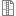# Examine Directly Proportional Relationships via a Lens of Artistic Creativity

Length: One 90-minute class period

The Metropolitan Nashville Arts Commission engaged two internationally-known artists, Thornton Dial and Lonnie Holley, to create site-specific public art works for the newly revitalized Edmondson Park (overseen by the Metropolitan Development and Housing Agency). This project honors William Edmondson, a native of Davidson County and a self-taught sculptor. Edmondson was the first African American artist to have a solo exhibition at the New York Museum of Modern Art (1937). Like Edmondson, Thornton Dial and Lonnie Holley are self-taught artists.

In this Mathematics Lesson, students will:

• Students will compare measurements of pieces created by both William Edmonson and Lonnie Holley in order to determine proportionality.
• The students will present their solutions to each other. The students will analyze and critique the solutions and provide constructive feedback.
• Students will be expected to explain which scale drawings are accurate/proportional based the equations that they derive.
• The students will be precise about labeling their tables and providing detail in relation to the construction of their equation.
• The students will look for the patterns in the problem in order to create the  structure of the equation.
• Exit slip will include review of new vocabulary as well as an opportunity to solve to problems in order to prove that students have mastered the day’s topic of direct proportionality.Full Lesson PlanLesson Materials

### Standards & Objectives

CCSS.Math.Content.7.RP.A.1
Compute unit rates associated with ratios of fractions, including ratios of lengths, areas and other quantities measured in like or different...
CCSS.Math.Content.7.RP.A.2
Recognize and represent proportional relationships between quantities.
CCSS.Math.Content.7.RP.A.3
Use proportional relationships to solve multistep ratio and percent problems. Examples: simple interest, tax, markups and markdowns, gratuities and...
GLE 0706.2.3
Develop an understanding of and apply proportionality.
GLE 0706.3.5
Understand and graph proportional relationships.
GLE 0706.3.6
Conceptualize the meanings of slope using various interpretations, representations, and contexts.
GLE 0706.4.2
Apply proportionality to converting among different units of measurements to solve problems involving rates such as motion at a constant speed.
GLE 0706.4.4
Understand and use ratios, derived quantities, and indirect measurements.
SPI 0706.1.3
Recognize whether information given in a table, graph, or formula suggests a directly proportional, linear, inversely proportional, or other nonlinear relationship.
SPI 0706.2.6
Express the ratio between two quantities as a percent, and a percent as a ratio or fraction.
SPI 0706.3.4
Interpret the slope of a line as a unit rate given the graph of a proportional relationship.
SPI 0706.3.5
Represent proportional relationships with equations, tables and graphs.
TSS.Math.7.RP.A.1
Compute unit rates associated with ratios of fractions, including ratios of lengths, areas, and other quantities measured in like or different units. For...
TSS.Math.7.RP.A.2
Recognize and represent proportional relationships between quantities.

Alignment of this item to academic standards is based on recommendations from content creators, resource curators, and visitors to this website. It is the responsibility of each educator to verify that the materials are appropriate for your content area, aligned to current academic standards, and will be beneficial to your specific students.

Learning objectives:

Clear Learning Targets:

• I can compute unit rates associated with ratios.
• I can recognize and represent proportional relationships between quantities.
• I can identify a table as being proportional or not proportional.
• I can use the proper formulas to identify proportional relationships.
• I can use slope formula.

Task Objectives (steps to reach mastery of clear learning targets):

• Determine whether two quantities are proportional by examining the relationship given in a table, graph, equation diagram, or as a verbal description.
• Identify the constant proportionality when presented with a proportional relationship in the form of a table, equation, or diagram.
• Write an equation that represents a proportional relationship.
• Use words to explain the relevance of a specific point on the graph of a proportional relationship,
• Including, but not limited to (0,0) and (1,r).
Essential and guiding questions:

Questioning: Planning to Illuminate Student Thinking:
Assessing questions:

• What can you tell me about the table? What do you see?
• Based on the measurements of William Edmonson’s piece, what can you tell me about the table?
• How do you know that your equation fits the pattern?

• How can ratios and proportional  relationships be used to determine unknown quantities (scaling up or down). What makes you think that?
• How can we determine the accuracy of a given scale? Why do you say that?

### Lesson Variations

Blooms taxonomy level:
Applying
Differentiation suggestions:

Scaffolding opportunities (to address learning challenges):

• The teacher will emphasize that students must read graphs as y per x, in addition to labeling the first two order pairs of all tables.
• The teacher will provide students with a variety of opportunities to encourage the learning of the equation for direct proportionality. These opportunities will include practice problems (converting tables to equation), direct application (applying skills to real world examples), and fun acronyms to assist students with the learning (example: the equation for direct is y= mx so an example memory device is “demarco young equals mr extraordinary-- to remember the order of the letters in the equation).
• The teacher will have students check to make sure that their equations work with all of the tables and revise as needed.

Opportunities to Differentiate Learning (explain how you address particular student needs by differentiating process, content, or product):

• The teacher will group students based on mastery of perquisite skills with groups ranging from 3-4 students. Students will be placed according to ability levels. The groups will remain the same for the duration of the lesson.
• Students who struggle with note-taking and keeping up with the pace of the lesson will be provided with graphic organizers.